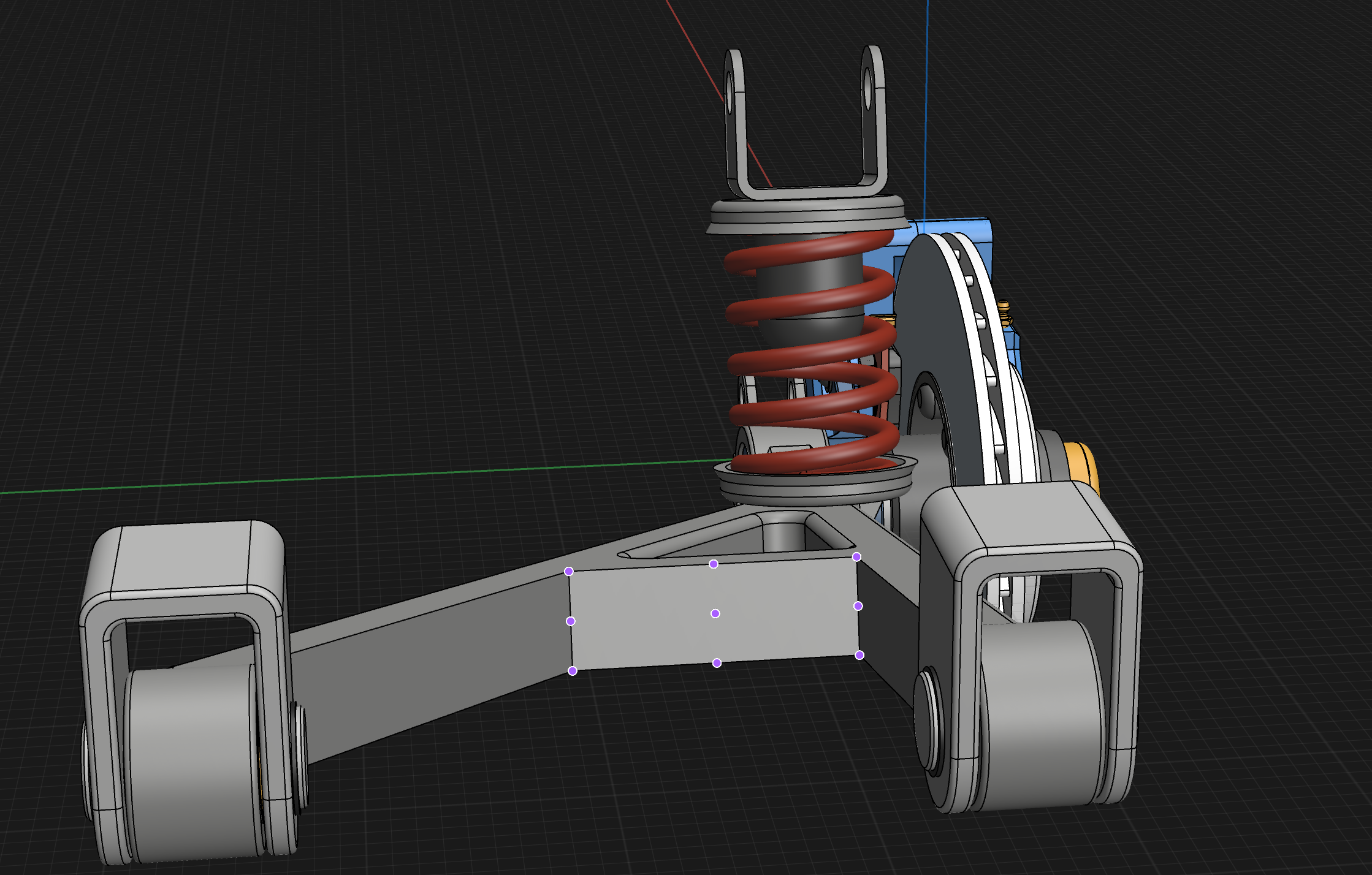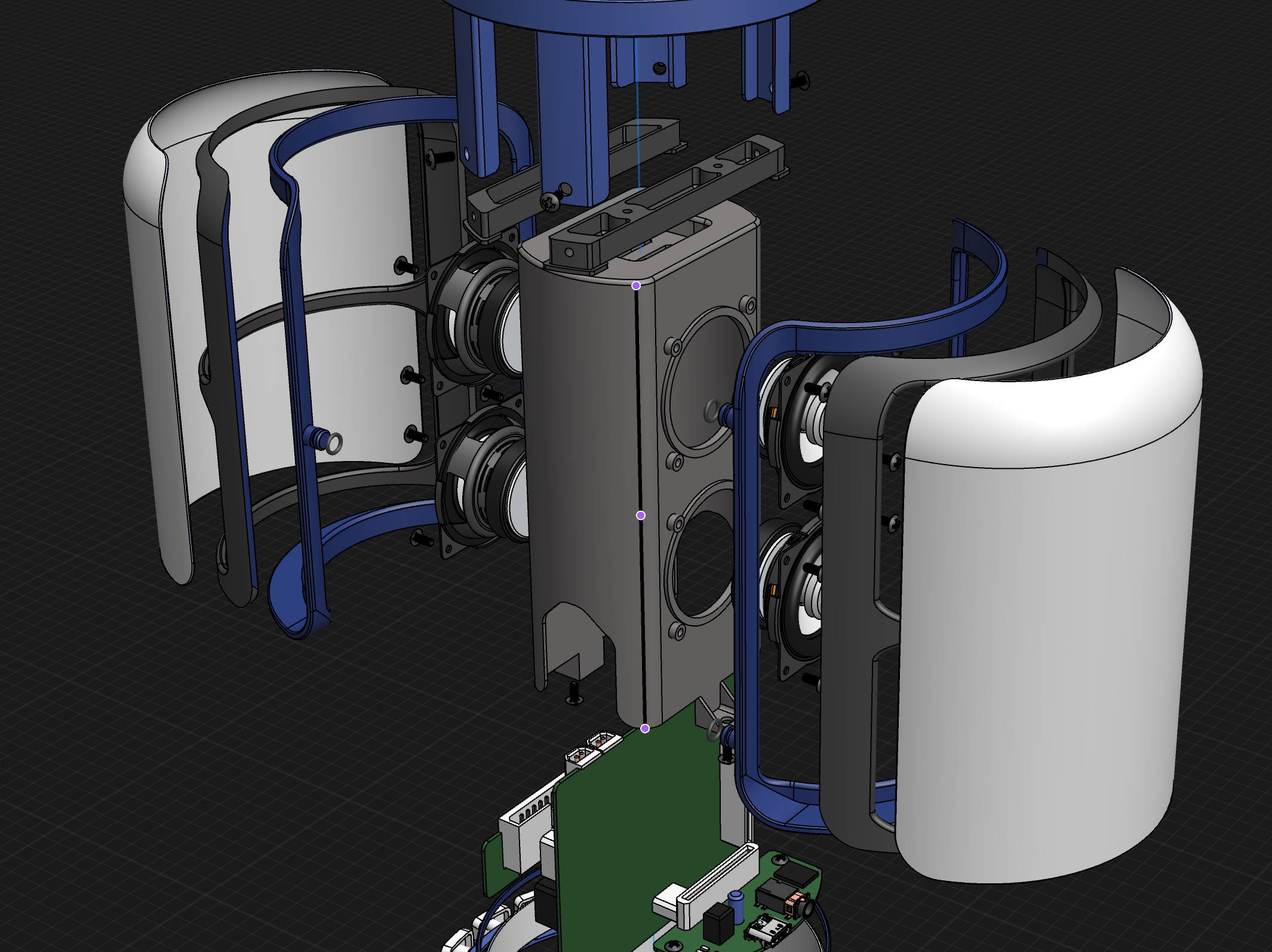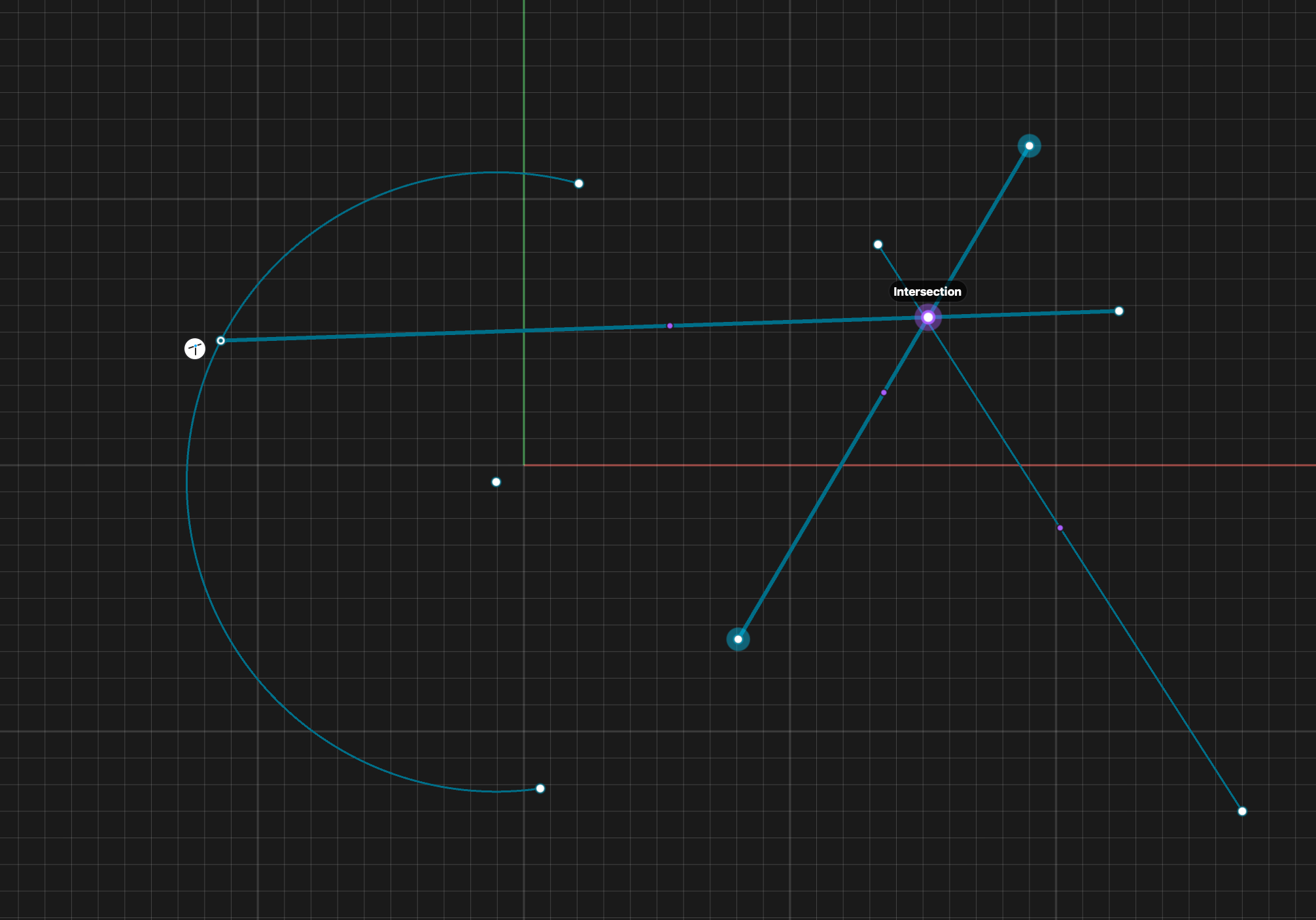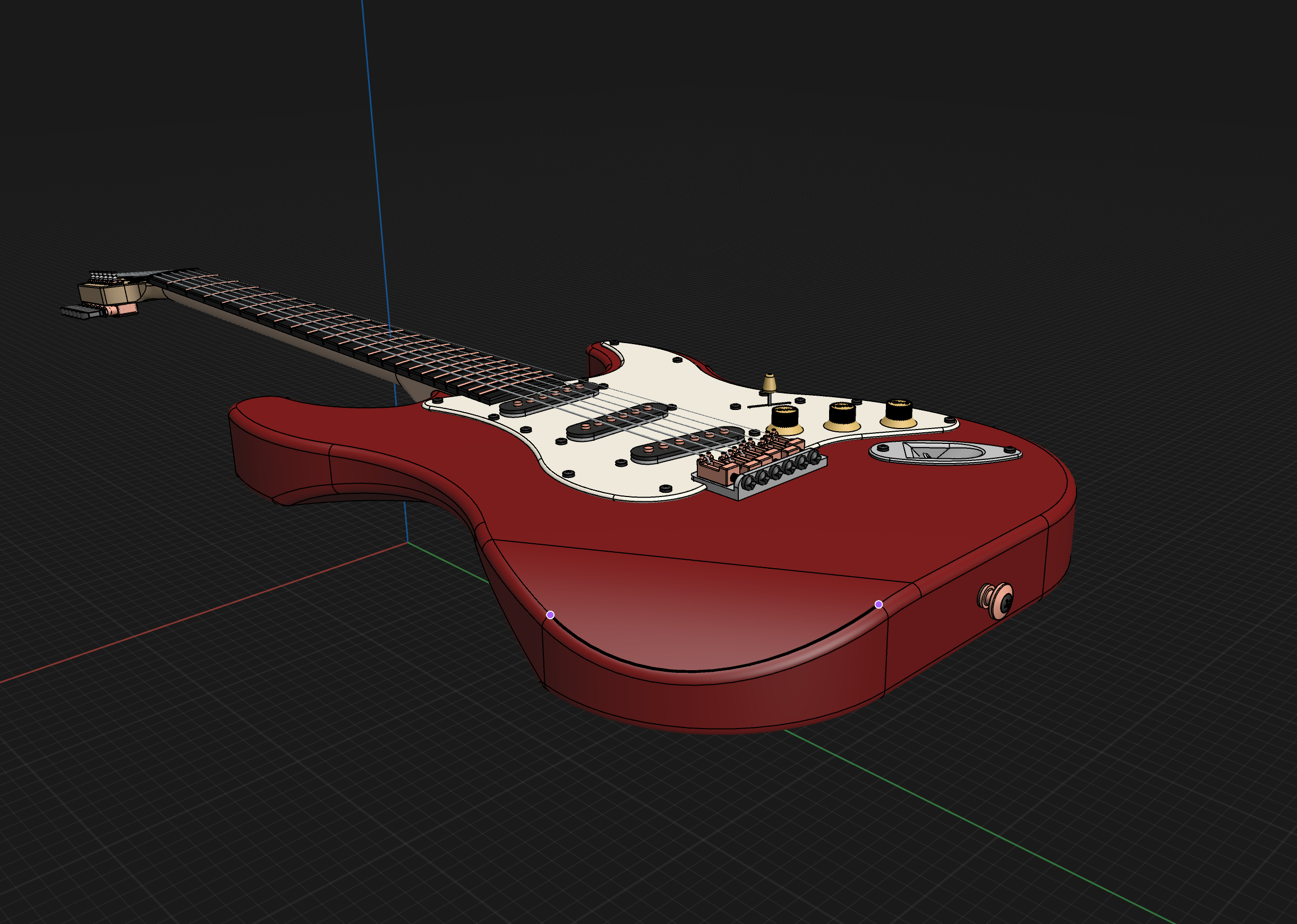# Notable points

Notable points, also known as snapping points or object snaps, are points to help you identify specific locations within sketches or bodies. These points are particularly useful when making measurements, using constraints, connecting sketches, or aligning 3D bodies. You can turn on and turn off the visibility of these points from the Snap To settings.

## Types of notable points

### Endpoints

Endpoints are found in linear, arc, and spline-based sketch elements or edges of bodies. Faces of 3D bodies inherit all the notable points from their bounding edges.

In this example, we have a selected rectangular face with four endpoints located at its four corners. These notable points are the endpoints of each edge, which they also share with the edges of their adjacent faces.### Midpoints

A midpoint is the middle point between two endpoints of a line segment. Midpoints can be found on linear sketch elements or edges.

In this example, the selected linear element has one midpoint and two endpoints.### Center points

Center points are points at the center of arc and circular edges, and planar rectangular faces. They can be found on cylindrical and conical faces of 3D bodies.

In this example, the selected arc element has one center point and two endpoints.### Intersection points

An intersection point is the point at which two lines meet or intersect. Intersection points can be found on linear sketch elements.

This example shows the intersection point at which the linear sketches meet.#### Tip

Splines, no matter how symmetrical, don’t have midpoints or center points. If these points are missing an item, check if the element has a measurable radius or diameter to make sure that it is not a spline.

Below is an example of a spline-based selection with two endpoints and no midpoint.